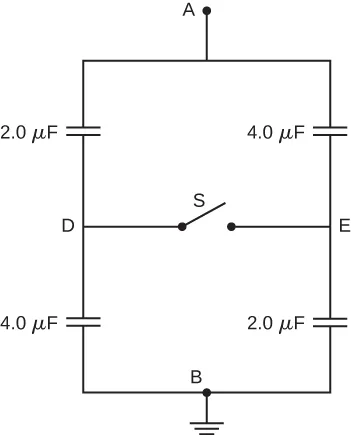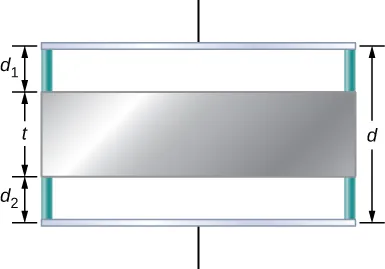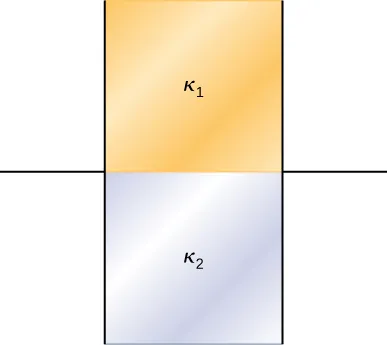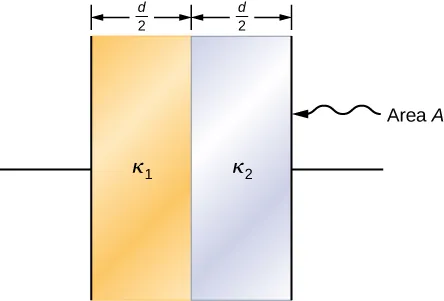University Physics Volume 2

# Challenge Problems

University Physics Volume 2Challenge Problems

### Challenge Problems

77.

A spherical capacitor is formed from two concentric spherical conducting spheres separated by vacuum. The inner sphere has radius 12.5 cm and the outer sphere has radius 14.8 cm. A potential difference of 120 V is applied to the capacitor. (a) What is the capacitance of the capacitor? (b) What is the magnitude of the electrical field at $r=12.6cmr=12.6cm$, just outside the inner sphere? (c) What is the magnitude of the electrical field at $r=14.7cmr=14.7cm$, just inside the outer sphere? (d) For a parallel-plate capacitor the electrical field is uniform in the region between the plates, except near the edges of the plates. Is this also true for a spherical capacitor?

78.

The network of capacitors shown below are all uncharged when a 300-V potential is applied between points A and B with the switch S open. (a) What is the potential difference $VE−VDVE−VD$ ? (b) What is the potential at point E after the switch is closed? (c) How much charge flows through the switch after it is closed?79.

Electronic flash units for cameras contain a capacitor for storing the energy used to produce the flash. In one such unit the flash lasts for 1/675 fraction of a second with an average light power output of 270 kW. (a) If the conversion of electrical energy to light is 95% efficient (because the rest of the energy goes to thermal energy), how much energy must be stored in the capacitor for one flash? (b) The capacitor has a potential difference between its plates of 125 V when the stored energy equals the value stored in part (a). What is the capacitance?

80.

A spherical capacitor is formed from two concentric spherical conducting shells separated by a vacuum. The inner sphere has radius 12.5 cm and the outer sphere has radius 14.8 cm. A potential difference of 120 V is applied to the capacitor. (a) What is the energy density at $r=12.6cmr=12.6cm$, just outside the inner sphere? (b) What is the energy density at $r=14.7cmr=14.7cm$, just inside the outer sphere? (c) For the parallel-plate capacitor the energy density is uniform in the region between the plates, except near the edges of the plates. Is this also true for the spherical capacitor?

81.

A metal plate of thickness t is held in place between two capacitor plates by plastic pegs, as shown below. The effect of the pegs on the capacitance is negligible. The area of each capacitor plate and the area of the top and bottom surfaces of the inserted plate are all A. What is the capacitance of this system?82.

A parallel-plate capacitor is filled with two dielectrics, as shown below. When the plate area is A and separation between plates is d, show that the capacitance is given by

$C = ε 0 A d κ 1 + κ 2 2 . C = ε 0 A d κ 1 + κ 2 2 .$83.

A parallel-plate capacitor is filled with two dielectrics, as shown below. Show that the capacitance is given by

$C = 2 ε 0 A d κ 1 κ 2 κ 1 + κ 2 . C = 2 ε 0 A d κ 1 κ 2 κ 1 + κ 2 .$84.

A capacitor has parallel plates of area $12cm212cm2$ separated by 2.0 mm. The space between the plates is filled with polystyrene. (a) Find the maximum permissible voltage across the capacitor to avoid dielectric breakdown. (b) When the voltage equals the value found in part (a), find the surface charge density on the surface of the dielectric.

Order a print copy

As an Amazon Associate we earn from qualifying purchases.